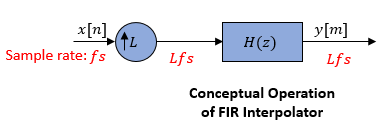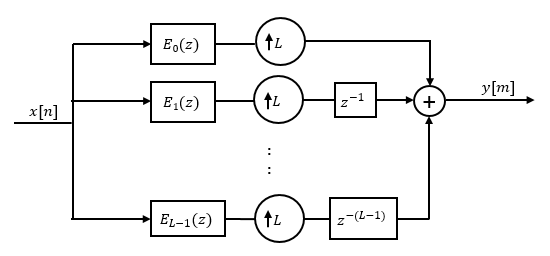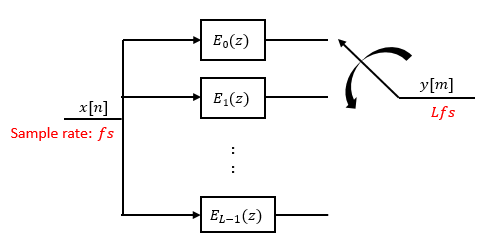Main Content

# dsp.FIRInterpolator

Polyphase FIR interpolator

## Description

The `dsp.FIRInterpolator` System object™ upsamples an input by the integer upsampling factor L along the first dimension. The FIR interpolator (as shown in the schematic) conceptually consists of an upsampler followed by an FIR anti-imaging filter, which is usually an approximation of an ideal band-limited interpolation filter. To design an anti-imaging filter, use the `designMultirateFIR` function.

The upsampler upsamples each channel of the input to a higher rate by inserting L–1 zeros between samples. The direct-form FIR filter that follows filters each channel of the upsampled data. The resulting discrete-time signal has a sample rate that is L times the original sample rate.Note that the actual object algorithm implements a polyphase structure, an efficient equivalent of the combined system depicted in the diagram. For more details, see Algorithms.

To upsample an input:

1. Create the `dsp.FIRInterpolator` object and set its properties.

2. Call the object with arguments, as if it were a function.

To learn more about how System objects work, see What Are System Objects?.

Under specific conditions, this System object also supports SIMD code generation. For details, see Code Generation.

## Creation

### Syntax

``firinterp = dsp.FIRInterpolator``
``firinterp = dsp.FIRInterpolator(interpFactor,num)``
``firinterp = dsp.FIRInterpolator(___,Name,Value)``

### Description

````firinterp = dsp.FIRInterpolator` returns an FIR interpolator, `firinterp`, which upsamples an input signal by a factor of 3 and applies an FIR filter to interpolate the output.```

example

````firinterp = dsp.FIRInterpolator(interpFactor,num)` returns an FIR interpolator with the integer-valued `InterpolationFactor` property set to `interpFactor` and the `Numerator` property set to `num`.```
````firinterp = dsp.FIRInterpolator(___,Name,Value)` returns an FIR interpolator object with each specified property set to the specified value. Enclose each property name in quotes. You can use this syntax with any previous input argument combinations.```

## Properties

expand all

Unless otherwise indicated, properties are nontunable, which means you cannot change their values after calling the object. Objects lock when you call them, and the `release` function unlocks them.

If a property is tunable, you can change its value at any time.

For more information on changing property values, see System Design in MATLAB Using System Objects.

Specify the integer factor, L, by which to increase the sampling rate of the input signal. The polyphase implementation uses L polyphase subfilters to compute convolutions at the lower sample rate. The FIR interpolator delays and interleaves these lower-rate convolutions to obtain the higher-rate output.

Data Types: `single` | `double` | `int8` | `int16` | `int32` | `int64` | `uint8` | `uint16` | `uint32` | `uint64`

FIR filter coefficient source, specified as either:

• `'Property'` –– The numerator coefficients are specified through the `Numerator` property.

• `'Input port'` –– The numerator coefficients are specified as an input to the object algorithm.

Specify the numerator coefficients of the FIR anti-imaging filter as the coefficients of a polynomial in z–1. The following equation defines the system function for a filter of length N+1:

`$H\left(z\right)=\sum _{n=0}^{N}b\left(n\right){z}^{-n}$`

The vector b = [b(0), b(1), …, b(N)] represents the vector of filter coefficients.

To act as an effective anti-imaging filter, the coefficients usually correspond to a lowpass filter with a normalized cutoff frequency no greater than the reciprocal of the `InterpolationFactor`. Use `designMultirateFIR` to design such a filter. More generally, any complex bandpass filter can be used. For an example, see Double the Sample Rate Using FIR Interpolator.

The filter coefficients are scaled by the value of the `InterpolationFactor` property before filtering the signal. To form the L polyphase subfilters, `Numerator` is appended with zeros if necessary.

#### Dependencies

This property applies when `NumeratorSource` is set to `'Property'`.

Data Types: `single` | `double` | `int8` | `int16` | `int32` | `int64` | `uint8` | `uint16` | `uint32` | `uint64`
Complex Number Support: Yes

### Fixed-Point Properties

Flag to use full-precision rules for fixed-point arithmetic, specified as one of the following:

• `true` –– The object computes all internal arithmetic and output data types using the full-precision rules. These rules provide the most accurate fixed-point numerics. In this mode, other fixed-point properties do not apply. No quantization occurs within the object. Bits are added, as needed, to ensure that no roundoff or overflow occurs.

• `false` –– Fixed-point data types are controlled through individual fixed-point property settings.

For more information, see Full Precision for Fixed-Point System Objects and Set System Object Fixed-Point Properties.

Rounding method for fixed-point operations. For more details, see rounding mode.

#### Dependencies

This property is not visible and has no effect on the numerical results when the following conditions are met:

• `FullPrecisionOverride` set to `true`.

• `FullPrecisionOverride` set to `false`, `ProductDataType` set to `'Full precision'`, `AccumulatorDataType` set to ```'Full precision'```, and `OutputDataType` set to `'Same as accumulator'`.

Under these conditions, the object operates in full precision mode.

Overflow action for fixed-point operations, specified as one of the following:

• `'Wrap'` –– The object wraps the result of its fixed-point operations.

• `'Saturate'` –– The object saturates the result of its fixed-point operations.

For more details on overflow actions, see overflow mode for fixed-point operations.

#### Dependencies

This property is not visible and has no effect on the numerical results when the following conditions are met:

• `FullPrecisionOverride` set to `true`.

• `FullPrecisionOverride` set to `false`, `OutputDataType` set to `'Same as accumulator'`, `ProductDataType` set to ```'Full precision'```, and `AccumulatorDataType` set to `'Full precision'`

Under these conditions, the object operates in full precision mode.

Data type of the FIR filter coefficients, specified as:

• `Same word length as input` –– The word length of the coefficients is the same as that of the input. The fraction length is computed to give the best possible precision.

• `Custom` –– The coefficients data type is specified as a custom numeric type through the `CustomCoefficientsDataType` property.

Word and fraction lengths of the coefficients data type, specified as an autosigned `numerictype` (Fixed-Point Designer) with a word length of 16 and a fraction length of 15.

#### Dependencies

This property applies when you set the `CoefficientsDataType` property to `Custom`.

Data type of the product output in this object, specified as one of the following:

• `'Full precision'` –– The product output data type has full precision.

• `'Same as input'` –– The object specifies the product output data type to be the same as that of the input data type.

• `'Custom'` –– The product output data type is specified as a custom numeric type through the `CustomProductDataType` property.

For more information on the product output data type, see Multiplication Data Types.

#### Dependencies

This property applies when you set `FullPrecisionOverride` to `false`.

Word and fraction lengths of the product data type, specified as an autosigned numeric type with a word length of 32 and a fraction length of 30.

#### Dependencies

This property applies only when you set `FullPrecisionOverride` to `false` and `ProductDataType` to `'Custom'`.

Data type of an accumulation operation in this object, specified as one of the following:

• `'Full precision'` –– The accumulation operation has full precision.

• `'Same as product'` –– The object specifies the accumulator data type to be the same as that of the product output data type.

• `'Same as input'` –– The object specifies the accumulator data type to be the same as that of the input data type.

• `'Custom'` –– The accumulator data type is specified as a custom numeric type through the `CustomAccumulatorDataType` property.

#### Dependencies

This property applies when you set `FullPrecisionOverride` to `false`.

Word and fraction lengths of the accumulator data type, specified as an autosigned numeric type with a word length of 32 and a fraction length of 30.

#### Dependencies

This property applies only when you set `FullPrecisionOverride` to `false` and `AccumulatorDataType` to `'Custom'`.

Data type of the object output, specified as one of the following:

• `'Same as accumulator'` –– The output data type is the same as that of the accumulator output data type.

• `'Same as input'` –– The output data type is the same as that of the input data type.

• `'Same as product'` –– The output data type is the same as that of the product output data type.

• `'Custom'` –– The output data type is specified as a custom numeric type through the `CustomOutputDataType` property.

#### Dependencies

This property applies when you set `FullPrecisionOverride` to `false`.

Word and fraction lengths of the output data type, specified as an autosigned numeric type with a word length of 16 and a fraction length of 15.

#### Dependencies

This property applies only when you set `FullPrecisionOverride` to `false` and `OutputDataType` to `'Custom'`.

## Usage

### Syntax

``y = firinterp(x)``
``y = firinterp(x,num)``

### Description

example

````y = firinterp(x)` interpolates the input signal `x` along the first dimension, and outputs the upsampled and filtered values, `y`.```
````y = firinterp(x,num)` uses the FIR filter, `num`, to interpolate the input signal. This configuration is valid only when the `'NumeratorSource'` property is set to `'Input port'`.```

### Input Arguments

expand all

Data input, specified as a vector or a matrix. A P-by-Q input matrix is treated as Q independent channels, and the System object interpolates each channel over the first dimension and generates a P*L-by-Q output matrix, where L is the interpolation factor.

This object supports variable-size input and does not support complex unsigned fixed-point inputs.

Data Types: `single` | `double` | `int8` | `int16` | `int32` | `int64` | `uint8` | `uint16` | `uint32` | `uint64` | `fi`
Complex Number Support: Yes

FIR filter coefficients, specified as a row vector.

#### Dependencies

This input is accepted only when the `'NumeratorSource'` property is set to `'Input port'`.

Data Types: `double`
Complex Number Support: Yes

### Output Arguments

expand all

FIR interpolator output, returned as a vector or a matrix of size P*L-by-Q, where L is the interpolation factor.

Data Types: `single` | `double` | `int8` | `int16` | `int32` | `int64` | `uint8` | `uint16` | `uint32` | `uint64` | `fi`
Complex Number Support: Yes

## Object Functions

To use an object function, specify the System object as the first input argument. For example, to release system resources of a System object named `obj`, use this syntax:

`release(obj)`

expand all

 `freqz` Frequency response of discrete-time filter System object `fvtool` Visualize frequency response of DSP filters `info` Information about filter System object `cost` Estimate cost of implementing filter System object `polyphase` Polyphase decomposition of multirate filter `generatehdl` Generate HDL code for quantized DSP filter (requires Filter Design HDL Coder) `impz` Impulse response of discrete-time filter System object `coeffs` Returns the filter System object coefficients in a structure
 `step` Run System object algorithm `release` Release resources and allow changes to System object property values and input characteristics `reset` Reset internal states of System object

## Examples

collapse all

Note: If you are using R2016a or an earlier release, replace each call to the object with the equivalent `step `syntax. For example, `obj(x) `becomes `step(obj,x)`.

Note: The `audioDeviceWriter` System object™ is not supported in MATLAB Online.

This example shows how to double the sampling rate of an audio signal from 22.05 kHz to 44.1 kHz, and play the audio.

```afr = dsp.AudioFileReader('OutputDataType',... 'single'); adw = audioDeviceWriter(44100); L = 2; num = designMultirateFIR(L,1); firInterp = dsp.FIRInterpolator(L,num); while ~isDone(afr) frame = afr(); y = firInterp(frame); adw(y); end pause(1); release(afr); release(adw);```

## Algorithms

The FIR interpolation filter is implemented efficiently using a polyphase structure.

To derive the polyphase structure, start with the transfer function of the FIR filter:

`$H\left(z\right)={b}_{0}+{b}_{1}{z}^{-1}+...+{b}_{N}{z}^{-N}$`

N+1 is the length of the FIR filter.

You can rearrange this equation as follows:

`$H\left(z\right)=\begin{array}{c}\left({b}_{0}+{b}_{L}{z}^{-L}+{b}_{2L}{z}^{-2L}+..+{b}_{N-L+1}{z}^{-\left(N-L+1\right)}\right)+\\ {z}^{-1}\left({b}_{1}+{b}_{L+1}{z}^{-L}+{b}_{2L+1}{z}^{-2L}+..+{b}_{N-L+2}{z}^{-\left(N-L+1\right)}\right)+\\ \begin{array}{c}⋮\\ {z}^{-\left(L-1\right)}\left({b}_{L-1}+{b}_{2L-1}{z}^{-L}+{b}_{3L-1}{z}^{-2L}+..+{b}_{N}{z}^{-\left(N-L+1\right)}\right)\end{array}\end{array}$`

L is the number of polyphase components, and its value equals the interpolation factor that you specify.

You can write this equation as:

`$H\left(z\right)={E}_{0}\left({z}^{L}\right)+{z}^{-1}{E}_{1}\left({z}^{L}\right)+...+{z}^{-\left(L-1\right)}{E}_{L-1}\left({z}^{L}\right)$`

E0(zL), E1(zL), ..., EL-1(zL) are polyphase components of the FIR filter H(z).

Conceptually, the FIR interpolation filter contains an upsampler followed by an FIR lowpass filter H(z).Replace H(z) with its polyphase representation.Here is the multirate noble identity for interpolation.Applying the noble identity for interpolation moves the upsampling operation to after the filtering operation. This move enables you to filter the signal at a lower rate.You can replace the upsampling operator, delay block, and adder with a commutator switch. The switch starts on the first branch 0 and moves in the counter clockwise direction, each time receiving one sample from each branch. The interpolator effectively outputs L samples for every one input sample it receives. Hence the sample rate at the output of the FIR interpolation filter is Lfs.## Extended Capabilities

### Fixed-Point ConversionDesign and simulate fixed-point systems using Fixed-Point Designer™.

Introduced in R2012a

Watch now